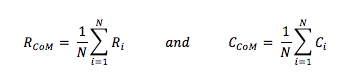Thursday, 8 March 2018

Extraction of centre of mass of a binary pattern is an important task in pattern recognition area in digital image processing domain. The pattern’s shape and size can be estimated using the radii profile that is computed around the centre of mass. The centre of mass of a binary pattern is computed using the first order moments of the pattern pixel’s Cartesian Coordinates. For all, very firstly the pattern is converted to a binary class i.e. pattern as in black on a white color background or vice versa. Black and white colors are attributed as 0 and 1 respectively (binary form) in digital image processing.
In matlab IDE, the image dimensions are taken as row x column. The pixel at (0,0) location is at top left corner of the image (refer to figure-1). An example pixel at location (3,7) is shown as F(3,7).
A grey color pattern of size 7×5 is shown in figure-2. It is converted to binary pattern as shown in figure-3. The grey pattern can be binarized using the Otsu algorithm. The equivalent binary pattern is shown in figure-3.